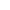# 卡尔曼滤波算法原理、五个公式、作用、局限性及应用场合卡尔曼全名RudolfEmilKalman，匈牙利数学家，1930年出生于匈牙利首都布达佩斯。1953，1954年于麻省理工学院分别获得电机工程学 士及硕士学位。1957年于哥伦比亚大学获得博士学位。我们现在要学习的卡尔曼滤波器，正是源于他的博士论文和1960年发表的论文 《ANewApproachtoLinearFilteringandPredictionProblems》（线性滤波与预测问题的新方法）。

X(k)=AX(k-1)+BU(k)+W(k)

Z(k)=HX(k)+V(k)

X(k|k-1)=AX(k-1|k-1)+BU(k)………..(1)

P(k|k-1)=AP(k-1|k-1)A’+Q(2)

X(k|k)=X(k|k-1)+Kg(k)(Z(k)-HX(k|k-1))(3)

Kg(k)=P(k|k-1)H’/(HP(k|k-1)H’+R)(4)

P(k|k)=（I-Kg(k)H）P(k|k-1)(5)

①卡尔曼滤波是一个算法，它适用于线性、离散和有限维系统。每一个有外部变量的自回归移动平均系统(ARMAX)或可用有理传递函数表示的系统都可以转换成用状态空间表示的系统，从而能用卡尔曼滤波进行计算。

②任何一组观测数据都无助于消除x(t)的确定性。增益K(t)也同样地与观测数据无关。

③当观测数据和状态联合服从高斯分布时用卡尔曼递归公式计算得到的是高斯随机变量的条件均值和条件方差，从而卡尔曼滤波公式给出了计算状态的条件概率密度的更新过程线性最小方差估计，也就是最小方差估计。